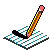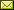PHYSICS@HORTONPhysics12

Physics 11

Math 11

NS Exams

Curriculum

Tips

Tutorials

McGraw Hill

Resources

Need it?

News

Enrichment

GiancoliIB Physics SL

April 2015 Notes

 April 1 Interpretation of Kinematics graphs (continued) Relative Motion & Frames of Reference Car in Snow Storm (from Penn State Animations for Physics & Astronomy) Relative Motion Compare Relative Motion in Different Frames (simulation) Graphs of Motion Review Booklet Notes April 2 Projectile Motion Video Analysis of Projectile Motion (Ball Toss ~ Physics Tool Kit) Video Analysis of Projectile Motion (Bird Toss in moving chair ~ Logger Pro) Notes April 7 More Projectile Motion Notes April 8 PRESCRIBED PRACTICAL ~ Measuring the acceleration due to gravity by timing a freefalling object (write up as DCP/CE) April 9 QUIZ (2.1 Kinematics) April 10 School Play April 13 Error Analysis Lab April 14 Work on Labs April 15 April 16 Forces (Weight/Normal Force/FBDs) Go over quiz BrainPop: Gravity BrainPop: Force What is Gravity? What is a Force? What Forces are Acting on You? Notes April 17 Forces (Resultant Forces/Balanced & Unbalanced Forces/Newton's First Law/Translational Equilibrium/Newton's Second Law) Notes Forces (Newton's Second Law) Forces & Motion: Basics (Tug of War & Acceleration Lab) (needs Java) Notes Forces (Friction) Forces in 1D (needs Java) The Physics Classroom The Calculator Pad Forces (Fluid Resistance) Notes April 20 work on Acceleration due to free fall April 21 Momentum & Impulse (Momentum, Impulse & Newton's Second Law/F-t graphs/Conservation of Linear Momentum) Momentum & Impulse More Momentum & Impulse Impulse Bouncing Ball Momentum & Collisions (PHYSCLIPS) Notes April 22 Momentum & Impulse (Conservation of Linear Momentum) Finish Popper Physics pHET Collision Lab (needs JAVA!!! ~ settings: 1D, check velocity vectors & momenta diagram, try 100%, 50%, 0% elasticity, try different mass combinations, try different velocities and look at the total mometum before & after collision) Notes April 23 Momentum & Impulse (Conservation of Linear Momentum) Notes April 27 Momentum & Impulse (Conservation of Linear Momentum) Notes Video: Momentum (Vector Addition) 2D Collisions (World-in-Motion video analysis) Another Example April 28 Momentum & Impulse (Newton's Third Law) Notes April 29 Work, power, and energy (Forms of energy, conservation of energy, work) BrainPop: Work BrainPop: Inclined Plane Notes

{subdirectory}